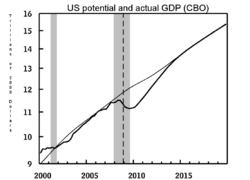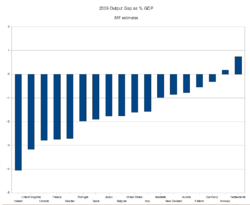# Output gap

﻿
Output gapPotential (light) and actual (bold) GDP estimates from the Congressional Budget Office. The difference between the two represents the GDP gap.IMF estimates of the 2009 output gaps as % of GDP by country

The GDP gap or the output gap is the difference between potential GDP and actual GDP or actual output. The calculation for the output gap is Y*–Y where Y* is actual output and Y is potential output. If this calculation yields a positive number it is called an inflationary gap and indicates the growth of aggregate demand is outpacing the growth of aggregate supply—possibly creating inflation; if the calculation yields a negative number it is called a recessionary gap—possibly signifying deflation. 

The percentage GDP gap is the actual GDP minus the potential GDP divided by the potential GDP.${(GDP_{actual} - GDP_{potential})}\over{GDP_{potential}}$.

## Okun's Law: The relationship between output and unemployment

Okun's Law is based on regression analysis of US data that shows a correlation between unemployment and GDP. Okun's law can be stated as: For every 1% increase in cyclical unemployment (actual unemployment - natural rate of unemployment), GDP will decrease by β%.

%Output gap = -β x %Cyclical unemployment

This can also be expressed as:

(Y-Y*) / Y* = -β(u-ū)

where:

• Y is actual output
• Y* is potential output
• u is actual unemployment
• ū is the natural rate of unemployment
• β is a constant derived from regression show the link between deviations from natural output & natural unemployment.

Wikimedia Foundation. 2010.

### Look at other dictionaries:

• Output Gap — An economic measure of the difference between the actual output of an economy and the output it could achieve when it is most efficient, or at full capacity. There are two types of output gaps: positive and negative. A positive output gap occurs… …   Investment dictionary

• Output-Gap — Der Begriff Produktionslücke (auch Outputlücke) bezeichnet die relative Abweichung des Bruttoinlandsprodukts BIP vom Produktionspotenzial PP, formal: . Siehe auch Auslastungsgrad …   Deutsch Wikipedia

• Output Gap — ⇡ Produktionslücke …   Lexikon der Economics

• GDP gap — The GDP gap or the output gap is the difference between potential GDP and actual GDP or actual output. The calculation for the output gap is Y Y* where Y is potential output and Y* is actual output or the natural level of output. If this… …   Wikipedia

• Potential output — In economics, potential output (also referred to as natural gross domestic product ) refers to the highest level of real Gross Domestic Product output that can be sustained over the long term. The existence of a limit is due to natural and… …   Wikipedia

• Spark-gap transmitter — A spark gap transmitter is a device for generating radio frequency electromagnetic waves. These devices served as the transmitters for most wireless telegraphy systems for the first three decades of radio (1887 ndash;1916) and the first… …   Wikipedia

• Comprehensible output — In the field of Second Language Acquisition, there are many theories about the most effective way for language learners to acquire new language forms. One theory of language acquisition is the Comprehensible Output Hypothesis. Developed by… …   Wikipedia

• Inflationary gap — An inflationary gap, in economics, is the amount by which the real Gross domestic product, or real GDP, exceeds potential GDP.cite journal last=Parkin first=Michael year= 2007 title=Economics, Level I CFA Program Curriculum volume=2… …   Wikipedia

• Inductive output tube — The inductive output tube or IOT is a variety of vacuum tube which evolved in the 1980s to meet increasing efficiency requirements for high power RF amplifiers. The primary commercial use of IOTs is in UHF television transmitters, where they have …   Wikipedia

• Okun Gap — A macroeconomic term that describes the situation when an economy s potential gross domestic product (GDP) differs from its actual gross domestic product. The gap can either be recessionary or inflationary, but will depend on the economy s… …   Investment dictionary